# Polyhedron group

The group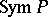of symmetries of a polytope (cf. Polyhedron)in an-dimensional Euclidean space, that is, the group of all motions ofwhich sendto itself. A polytopeis called regular ifacts transitively on the set of its "flag set of a polytopeflags" , that is, collections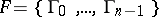whereis a-dimensional closed face and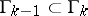. The group of symmetries of a regular polytope is generated by reflections (see Reflection group). Its fundamental domain is a simplicial conewhose vertex is the centre of the polytope, and whose edges pass through the centres of the faces constituting some flag. By the same token the generating reflections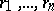of the grouphave a natural enumeration: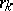is the reflection relative to the hyperplane boundingwhich does not pass through the centre of the face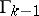. The generatorsand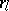commute for, and the order ofis equal to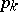— the number of-dimensional (or-dimensional) faces of the polytope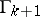containing the face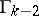(if it is assumed that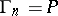and). The sequence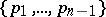is called the Schläfli symbol of the polytopes. The three-dimensional regular polytopes (Platonic solids) have the following Schläfli symbols: the tetrahedron, the cube, the octahedron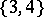, the dodecahedron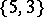, and the icosahedron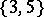.

The Schläfli symbol determines a regular polytope up to a similarity. Reversal of a Schläfli symbol corresponds to transition to the reciprocal polytope, whose vertices ly at the centres of the-dimensional faces of. Reciprocal polytopes have the same symmetry group.

All possible Schläfli symbols of regular polytopes can be obtained from the classification of finite reflection groups, by selecting those with a linear Coxeter graph. For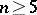there are only 3 regular polytopes in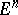: the simplex, the cube and the polytope reciprocal to the cube (the analogue of the octahedron). Their Schläfli symbols are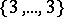,and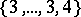. In-dimensional space there are 6 regular polytopes: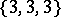,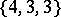,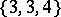,,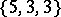, and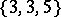.

Each face of a regular polytopeis also a regular polytope, the Schläfli symbol of which is the initial segment of the Schläfli symbol of. For example, a-dimensional face of the polytopehas the Schläfli symbol, that is, it is a dodecahedron.

How to Cite This Entry:
Polyhedron group. E.B. Vinberg (originator), Encyclopedia of Mathematics. URL: http://encyclopediaofmath.org/index.php?title=Polyhedron_group&oldid=12575
This text originally appeared in Encyclopedia of Mathematics - ISBN 1402006098